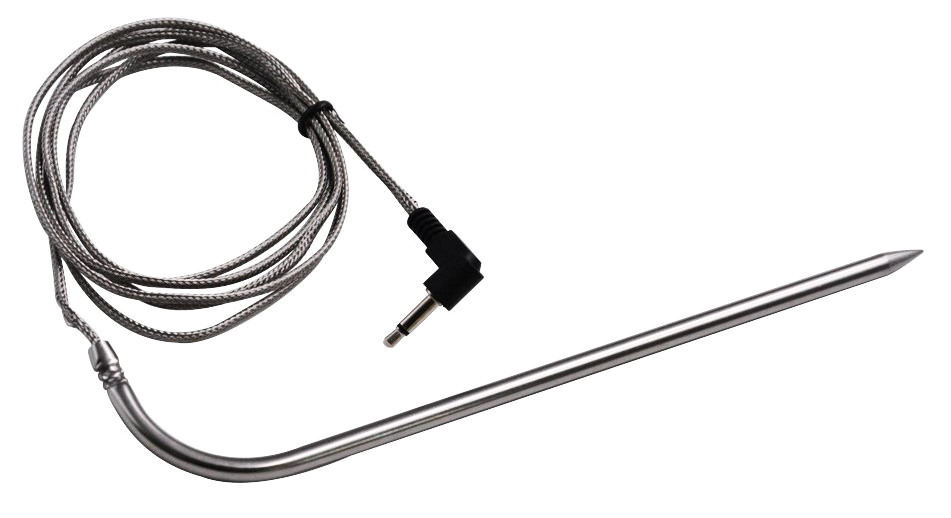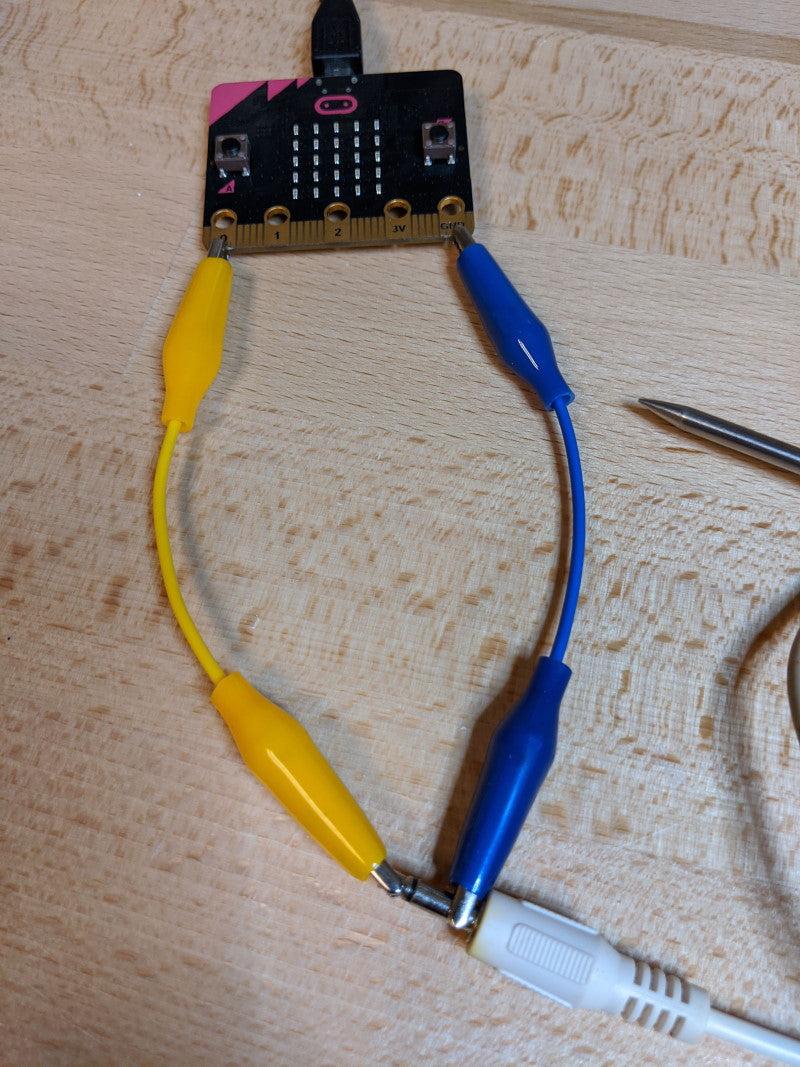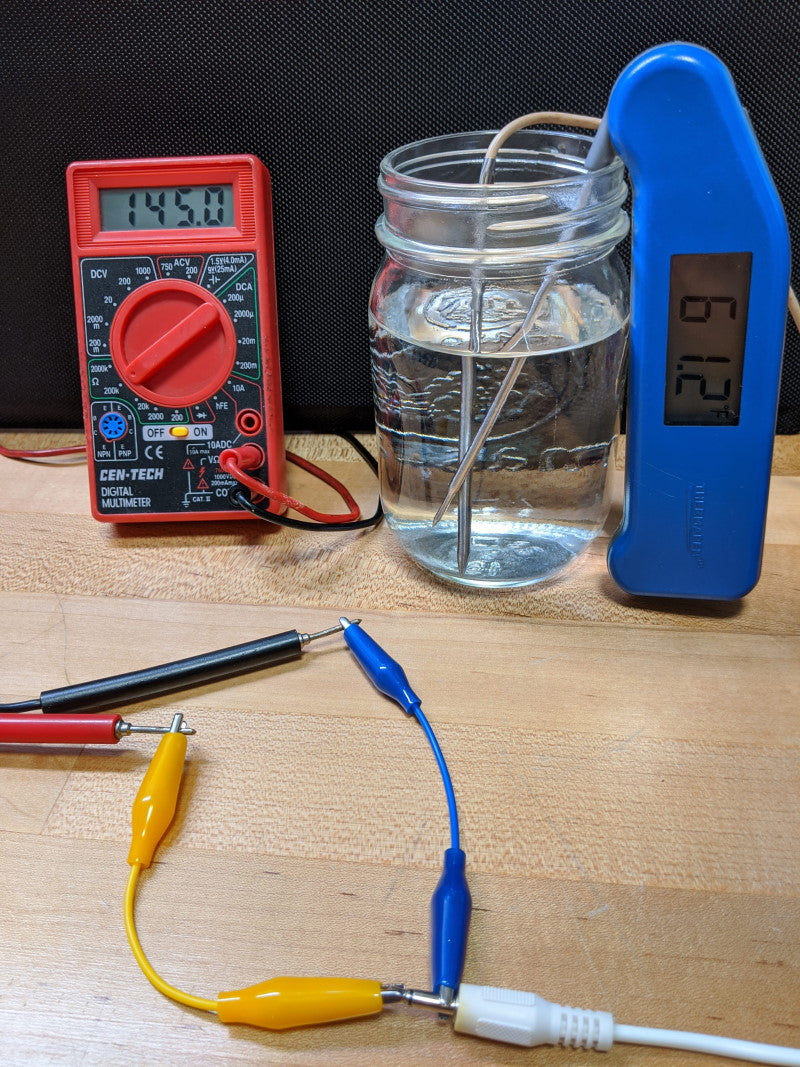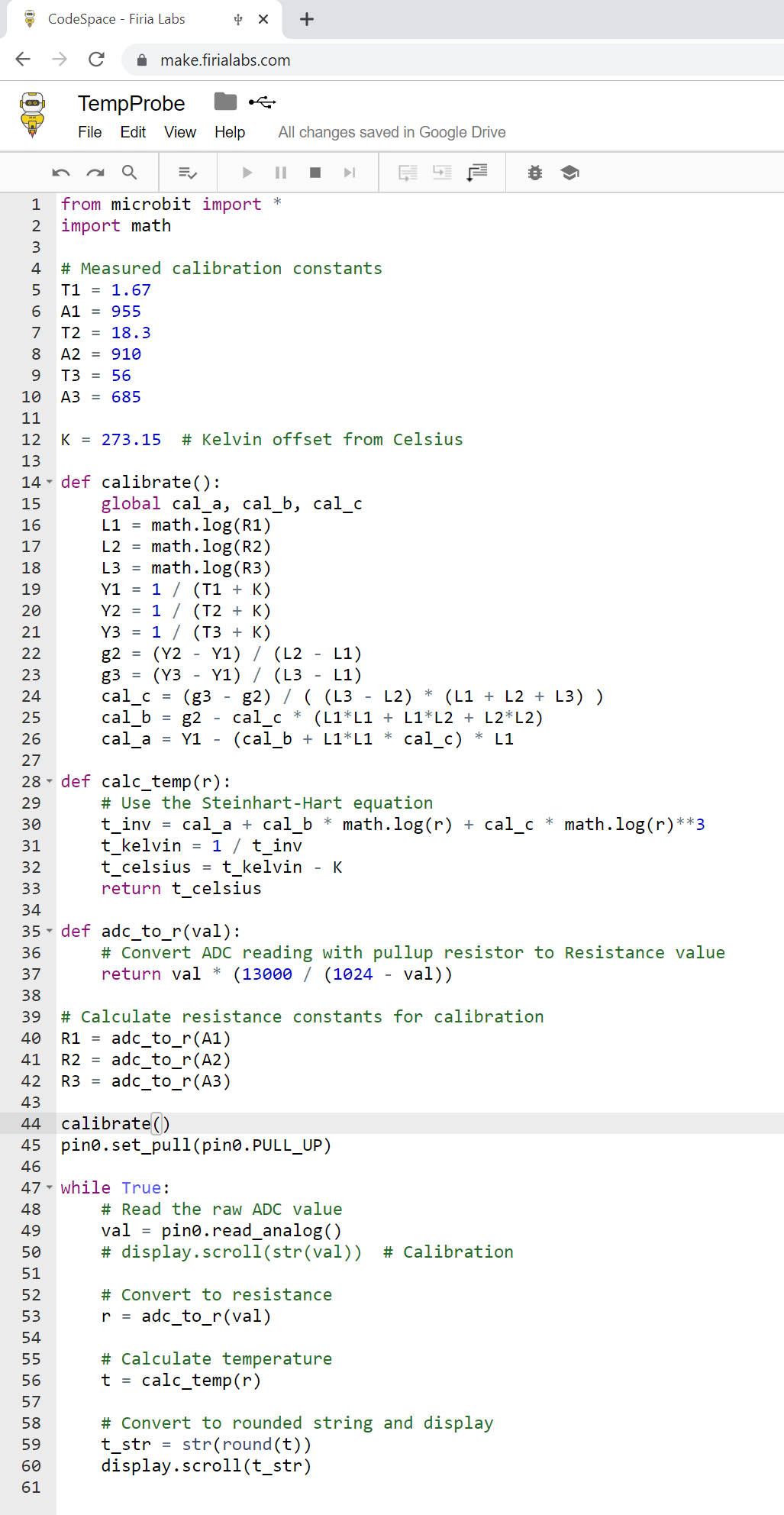×

We will use this information to send you occasional updates.
Firia Labs will never share your information with third parties.

# Kitchen Thermometer - Python likes Turkey a micro:bitAs we enter the final week of no blocks November, we're bringing you a practical project that might come in very handy for roasting your favorite seasonal dish. And if turkey's not your thing, there are countless uses for this project, culinary and otherwise!

Check out the video demo of this project here: https://youtu.be/di33GfSoQWc

THE COMMON THERMISTORIf you've been through our JumpStart Python projects, the basics of this code will be very familiar to you! Your JumpStart kit included a thermistor for measuring external temperatures. It turns out that common kitchen probe thermometers use exactly the same thermistor technology!You may already have one of these like the one shown here, but if not they can be purchased online for around \$10. Search for replacement probes.  I happened to have an old one sitting in a kitchen drawer, the cheap display unit it had once plugged into having long since stopped working. Wouldn't it be cool to breathe life back into this thing?!

CONNECTING A THERMISTOR TO THE MICRO:BIT

In JumpStart you used a discrete thermistor component. Connecting a kitchen probe is just as easy. The two pins of the device are connected to the contacts of the 1/8" plug at the end of the cable. For the code below, I connected to pin0 and GND.CALIBRATION

A thermistor is a variable resistor whose value changes based on temperature. Common thermistors are NTC devices, which stands for "Negative Temperature Coefficient". That means that as the temperature rises, the resistance value decreases. Different thermistors respond differently based on their materials and construction, so you'll need to calibrate yours to get an accurate temperature reading. To do this, you need another thermometer to use as a standard of measurement.(Ohmmeter is optional - see below to use the micro:bit instead!)

Calibrating for an accurate temperature measurement is pretty simple. You just need to take three measurements at different temperatures at the high, low, and medium levels of the range you're interested in measuring. I used a glass of ice water, room temp water, and hot tap-water for my three calibration points. A standard method for accurate thermistor calculations is the Steinhart-Hart Equation.  It uses three coefficients that are calculated from the three measured calibration points. You could use an Ohmmeter as shown in the picture above to measure the resistance for the R1, R2, and R3 resistances needed. Search for Steinhart Hart Calculator and you'll find some online forms that can calculate the coefficients for you (cal_a, cal_b, cal_c). Once you have those three coefficients, the Python code to get the temperature in Celsius based on measured resistance is:

``````def calc_temp(r):
# Use the Steinhart-Hart equation
t_inv = cal_a + cal_b * math.log(r) + cal_c * math.log(r)**3
t_kelvin = 1 / t_inv
t_celsius = t_kelvin - 273.15
return t_celsius
``````

MEASURING RESISTANCE WITH THE MICRO:BIT

You can use the built-in pullup resistor feature of the micro:bit to help measure the resistance of the thermistor. As we mentioned our the Buttons and Switches article, the pullup resistance is about 13k Ohms. The Python code to convert an ADC reading to resistance with that pullup enabled is:

``````def adc_to_r(val):
return val * (13000 / (1024 - val))
``````

With this code, you can use the micro:bit to measure the three calibration values as raw ADC values. Then in your code you can convert those to resistances (R1, R2, R3) and run the calibration calculation.

CALCULATING THE COEFFICIENTS

The Python code to calculate the three coefficients is as follows. Note that the calibration temperatures are measured in Celsius, and converted to Kelvin using the constant K = 273.15.

``````def calibrate():
global cal_a, cal_b, cal_c
L1 = math.log(R1)
L2 = math.log(R2)
L3 = math.log(R3)
Y1 = 1 / (T1 + K)
Y2 = 1 / (T2 + K)
Y3 = 1 / (T3 + K)
g2 = (Y2 - Y1) / (L2 - L1)
g3 = (Y3 - Y1) / (L3 - L1)
cal_c = (g3 - g2) / ( (L3 - L2) * (L1 + L2 + L3) )
cal_b = g2 - cal_c * (L1*L1 + L1*L2 + L2*L2)
cal_a = Y1 - (cal_b + L1*L1 * cal_c) * L1
``````

WRAP-UP AND REMIX IDEAS

We're going for maximum "style points" in the code below, doing the calibration AND the measurements right on the micro:bit. The neat thing is, this is a pretty accurate little measuring tool! And if you've worked through the Temperature Sensor project in JumpStart Python, you'll know exactly how to turn this into a wireless thermometer - you could even add an alarm when the temperature reaches a desired level!